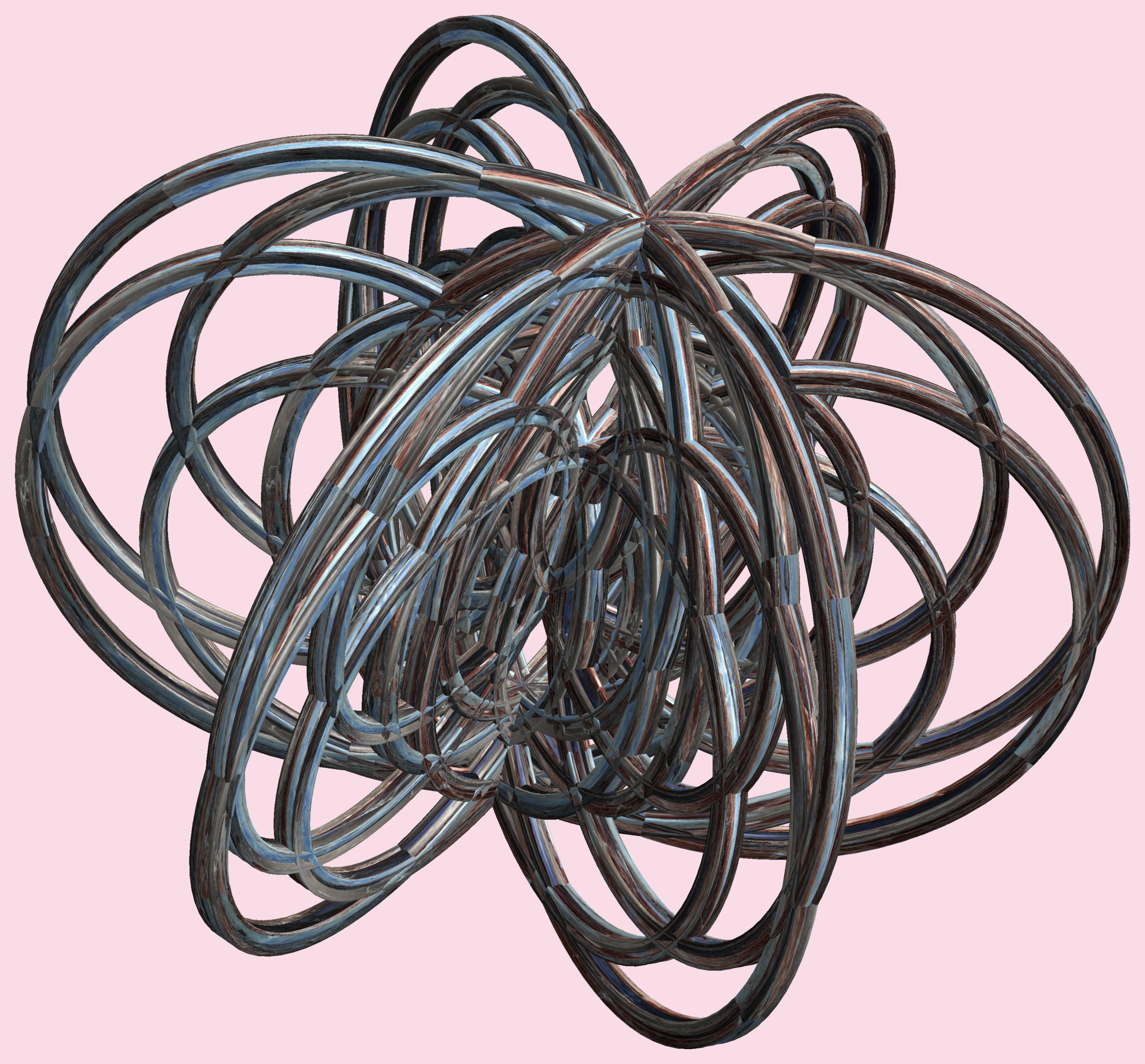# 6 Tori or 3D Lissajous figures - Mathematica / ６個の輪環(りんかん)または立体(りったい)リサージュ図形(ずけい)

###### Related for 6 Tori or 3D Lissajous figures - Mathematica / ６個の輪環(りんかん)または立体(りったい)リサージュ図形(ずけい)6 tori or 3d lissajous figures - mathematica / ６個の輪環(りんかん)または立体(りったい)リサージュ図形(ずけい) free stock photo

(* === Following code of Mathematica 8 generates this image. === *)

a1 = 20; (* center hole size of a torus *)

a2 = 20; (* center hole size of a torus *)

b1 = 4; (* the number of cross *)

b2 = 5; (* the number of cross *)

b3 = 3; (* the number of waves *)

b4 = 2; (* the number of waves *)

c1 = 12; (* distance from the center of rotation *)

c2 = 0; (* distance from the center of rotation *)

d = 6; (* the number of tori *)

h1 = 1; (* width of a torus *)

h2 = 10; (* width of a torus *)

h3 = 0; (* height of a torus *)

h4 = 1; (* height of a torus *)

SetOptions[

ParametricPlot3D, PlotRange -> Full, Mesh -> None, Boxed -> False, Axes -> False,

PlotPoints -> 500, ImageSize -> 3000, Background -> RGBColor[{250, 220, 230}/255],

PlotStyle -> Directive[Specularity[White, 90], Texture[Import["D:/tmp/942.jpg"]]],

TextureCoordinateFunction -> ({#4, #5 b1 Pi} &), Lighting -> "Neutral"];

x = (a1 - h1 Cos[b3 t] + h2 Cos[b1 s]) Cos[b2 s + Pi/b1] + c1;

y = (a2 - h1 Cos[b3 t] + h2 Cos[b1 s]) Sin[b2 s + Pi/b1] + c1;

z = h3 Cos[b1 s] + h4 Sin[b4 t] + c2;

rm = Table[{x, y, z}.RotationMatrix[2 Pi (i/d), {1, 0, 0}], {i, d}];

ParametricPlot3D[rm, {t, 0, 2 Pi}, {s, 0, 2 Pi}]

(*---

Another shapes and colors

*)

Free high resolution images 6 tori or 3d lissajous figures - mathematica / ６個の輪環(りんかん)または立体(りったい)リサージュ図形(ずけい), 3d, テクスチャ, とーらす, どーなつ, もよう.
Licence: Creative Commons - CC0. Photos transferred to the public domain from Creative Commons.

Comments:

## Related premium images287
0

### Flickr (Public Domain)

9174 images